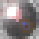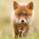1764 views
1764
small update: added ability to setup stage 2 length period.
```study(title="[RS]Vector Flow Channel V1", shorttitle="[RS]VFC.V1", overlay=true)
decay = atr(10)*0.005
stage1 = input(5)
stage2 = input(10)
stage3 = input(20)
stage1length = input(100)
stage2length = stage1length + input(100)
pretopvector01 = nz(topvector01, close)
topvector01 = close >= pretopvector01 ? close : pretopvector01 - (decay * topvector01finalcounter)
topvector01counter = n - valuewhen(close >= topvector01, n, 0)
topvector01finalcounter = topvector01counter <= stage1length ? stage1 : topvector01counter <= stage2length ? stage2 : stage3

prebotvector01 = nz(botvector01, close)
botvector01 = close <= prebotvector01 ? close : prebotvector01 + (decay * botvector01finalcounter)
botvector01counter = n - valuewhen(close <= botvector01, n, 0)
botvector01finalcounter = botvector01counter <= stage1length ? stage1 : botvector01counter <= stage2length ? stage2 : stage3

pt = plot(topvector01, color=(topvector01 == close ? na : black))
pb = plot(botvector01, color=(botvector01 == close ? na : black))
fill(pt, pb, color=blue, transp=90)
```RicardoSantos
Thanks, this is actually very useful for me. Much more than just chart art.RicardoSantos
no offense, but how is it based on regression? there is no regression computation in the code.isomorph
my apologies, poorly used term :s
it would likely make more sense as mean regression.RicardoSantos
ok no worries. could you please explain a little more about the origin of the algorithm? is this something you found on some site like stockcharts.com or investopedia? or is it your idea and if so, what is the main rationale behind it?

i ask because i am puzzled by this notion of using atr as a decay factor. in other words, why? why think of atr as 'decay'?isomorph
not at all, is mostly inspired by sar and gann fan's visual appearance, the rationale is the perception of price decay over time.

the atr was just a means to a end, it could be very well an input, pip or other unit of measurement.